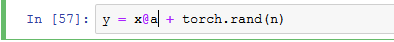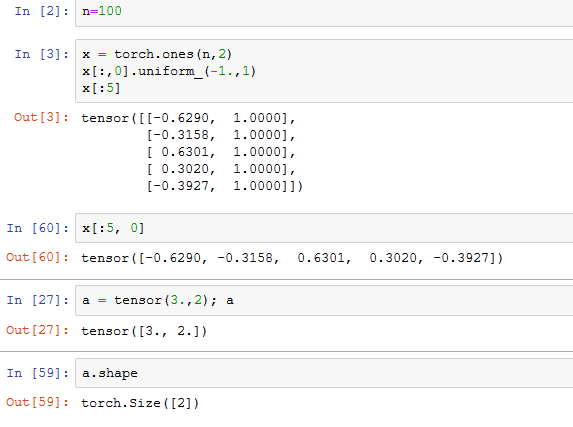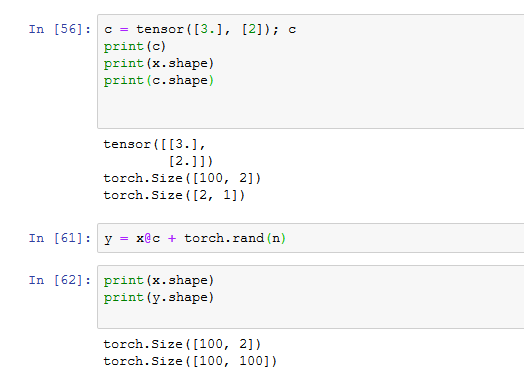# Understanding the "@" function

I am trying to understand matrix multiplication using the “@” function used in lecture 2.

Here is the equation that we are multiplying:Here is the code that defines the tensor (vector) a in the notebook:What confused me is that we are matrix multiplying x of shape (100,2) by a with shape (1,2). This should be undefined. But the shape of a is actually 2, not (1,2). I think this is called a “zero rank array”, and I understand from other courses that the behaviour can be a bit unpredictable. So my first question is how does this mutiplication work? Does numpy/pytorch just reconfigure the array to (2,1)?

I then tried to create my own row vector of shape (2,1) and multiplied it.X has shape (100, 2). c has shape (2, 1). Therefore X@c should have shape (100, 1). But it has shape (100, 100).

I obviously fundamentally misunderstand something about the @ function for matrix multiplication. Can someone help me out?

1 Like

Hi Mark. Please check the shapes of x@c and torch.rand(n) independently. You’ll probably need to learn about Python broadcasting. HTH, Malcolm

1 Like

@Pomo beat me to it, but thougt I’d post this anywayThe reason why `y = x@c + torch.rand(n)` has shape `(100, 100)` is a bit tricky: it’s because of what’s called “broadcasting” in the numpy/pytorch world.

You’re correct that `x@c` has shape `(100, 1)`. But you then add to it `torch.rand(n)`, which has shape `(100,)`. That’s a different shape than `x@c`. You might then reasonably wonder what should happen; the answer to this question (at least in numpy/pytorch etc.) is given by broadcasting.

The rule in this situation is that when you try to add a `(100, 1)` with a `(100,)`, broadcasting does two things: the `(100, 1)` shape changes to `(100, 100)` by copying (at least conceptually) each of those 1-element tensors 100 times; and then the `(100,)` shape gets nested to `(1, 100)`, and then that single 100-element tensor gets “copied” 100 times to get the shape `(100, 100)`. Now the two things we’re trying to add together have the same shape, and addition happens just the way you’d expect it to.

3 Likes

Thanks for the replies. I’m actually familiar with broadcasting. I’m coming over from Andrew Ng’s AI course where it was used a lot. What I’m not familiar with (because Andrew Ng always made a point that you should avoid them) is the behaviour of these zero rank arrays of shape (n, ).

I suppose if I use torch.rand(n,1) the result would be shape (100, 1)?

My larger question, though, is how these seemingly mismatched matrices are mutiplied together? If I multiply a tensor of shape (100, 2) with a tensor of shape (1,2), that is undefined, correct?

From your answer, I surmise that multiplying shape (100,2) with vector “a” of shape (2,) changes a to vector of shape (2, 1)? Do I have that right?

I get broadcasting when adding (100, 4) with (100, 1), for example. But I don’t know how to predict behaviour of arrays of shape (n,). Can you recommend a source?

Thanks again for the help.

1 Like

IMHO, the best source to develop understanding of PyTorch is to experiment directly in Jupyter.

My own internal model for matrix multiply says that the inner dimensions “cancel”. Therefore shapes [100,2] @  would yield shape . It would be logically consistent: that bias comes from having started my coding life with APL. But I have not actually done the experiment with PyTorch.

1 Like